# Squirrels

The squirrels discovered a bush with hazelnuts. The first squirrel plucked one nut, the second squirrel two nuts, the third squirrel three nuts. Each new squirrel always tore one nut more than the previous squirrel. When they plucked all the nuts from the bush, they divided the nuts so that each squirrel received six nuts. How many squirrels tore nuts?

n =  11

### Step-by-step explanation: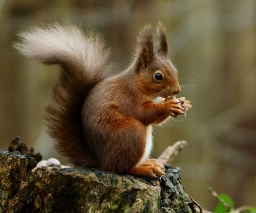Did you find an error or inaccuracy? Feel free to write us. Thank you!Tips to related online calculators
Looking for help with calculating arithmetic mean?
Looking for a statistical calculator?
Do you have a linear equation or system of equations and looking for its solution? Or do you have a quadratic equation?

#### You need to know the following knowledge to solve this word math problem:

We encourage you to watch this tutorial video on this math problem:

## Related math problems and questions:

• Three friends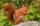Three friends squirrels together went to collect hazelnuts. Zrzecka he found more than twice Pizizubka and Ouska even three times more than Pizizubka. On the way home they talked while eating and was cracking her nuts. Pizizubka eaten half of all nuts whi
• Nuts, girl and boys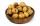Milena collected fallen nuts and called a bunch of boys let them share. She took a condition: the first boy takes one nut and tenth of the rest, the second takes 2 nuts and tenth new rest, the third takes 3 nuts and tenth new rest and so on. Thus managed
• NutsPeter has 49 nuts. Walnuts have 3 more than hazelnuts and hazelnut 2 over almonds. Determine the number of wallnuts, almonds and hazelnuts.
• Three drivers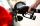Three driversdriving the same direction found that they have same amounth of gasoline. The first is enough to go 6 km, 4 km second and third 3km. Gasoline they divided so all three just drove to the nearest petrol station. How many km away was a petrol st
• Three workers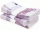The three workers received € 2,850 together for the work done. They divided them according to the time worked so that the first received 20% less than the second and the third € 50 more than the second. How much EUR did each worker receive?
• 3 masonsThree masons received 7,700 CZK. The second half received 1/2 more than the first and third twice more than the second mason. How much they each got crowns?
• Pine's forest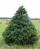There were so many pines in the forest that they were sequentially numbered 1, 2, 3,..., would use three times more digits than the pine trees alone. How many pine trees were there in the forest?
• The nuts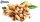There are 159 nuts in two bags, 15 more in the first than in the second, how many nuts are in the bags?
• Four pupilsFour pupils divided $1485 so that the second received 50% less than the first, the third 1/2 less than a fourth, and fourth$ 154 less than the first. How much money had each of them?
• Three workersThe company rewarded three workers CZK 9200, and the money was divided by the work they have done. The first worker to get twice than the second, the second three times more than the third. How much money did each worker receive?
• Three numbersFind three numbers so that the second number is 4 times greater than the first and the third is lower by 5 than the second number. Their sum is 67.
• Six-digit primesFind all six-digit prime numbers that contain each one of digits 1,2,4,5,7, and 8 just once. How many are they?
• AM of three numbersThe number 2010 can be written as the sum of 3 consecutive natural numbers. Determine the arithmetic mean of these numbers.
• Z9–I–4 MO 2017Numbers 1, 2, 3, 4, 5, 6, 7, 8 and 9 were prepared for a train journey with three wagons. They wanted to sit out so that three numbers were seated in each carriage and the largest of each of the three was equal to the sum of the remaining two. The conduct
• Sweets, candyGrandfather gave out sweets to four children. At the last moment, two more children came, so in order to have them all the same, each of the four children would receive four candies less than they would have received if they had not. How much did my grand
• Three excursions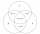Each pupil of the 9A class attended at least one of the three excursions. There could always be 15 pupils on each excursion. Seven participants of the first excursion also participated in the second, 8 participants of the first excursion, and 5 participan
• Scouts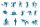Three scouts went on a three day trip. The second day went 4 km more than the first day. The third day went two times less than the first day. They went along 54 km. How many kilometers went every day together?